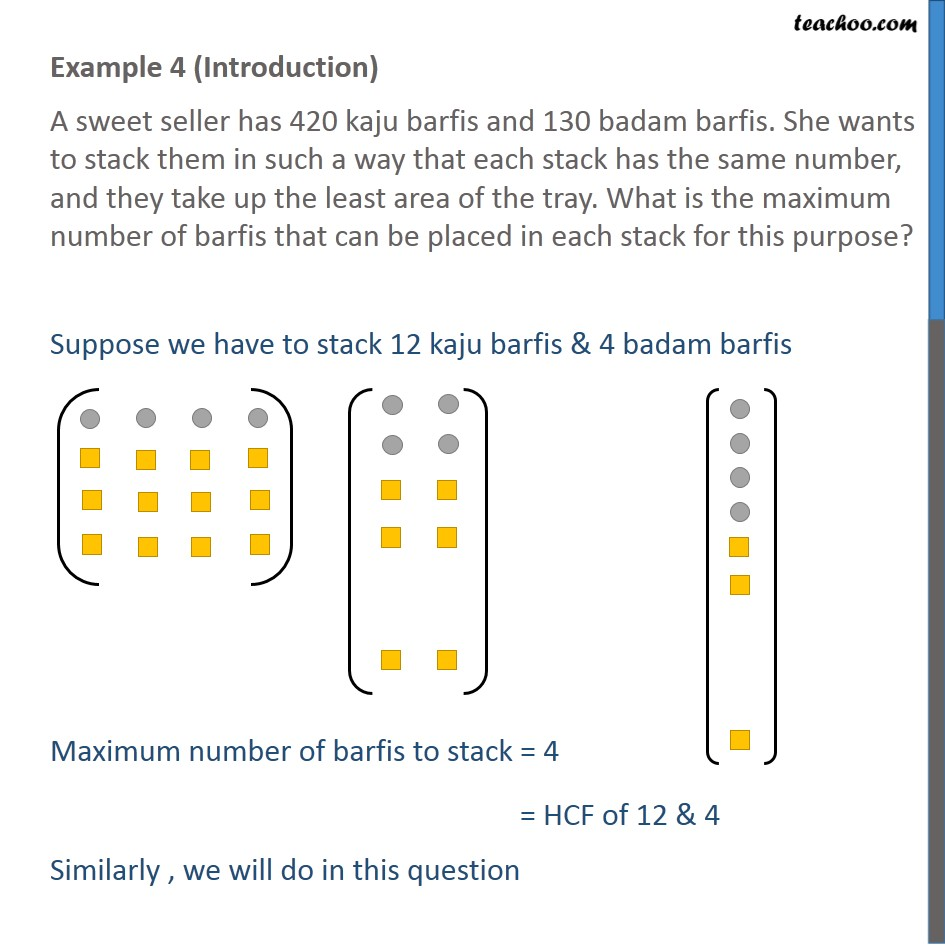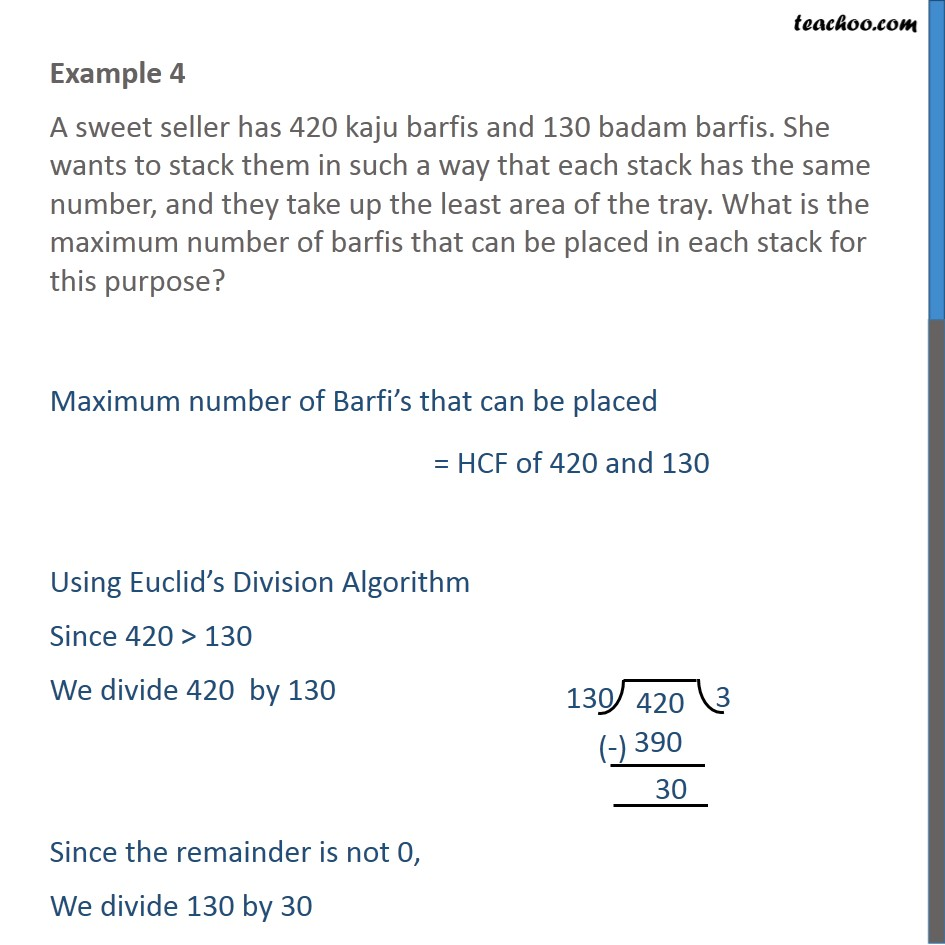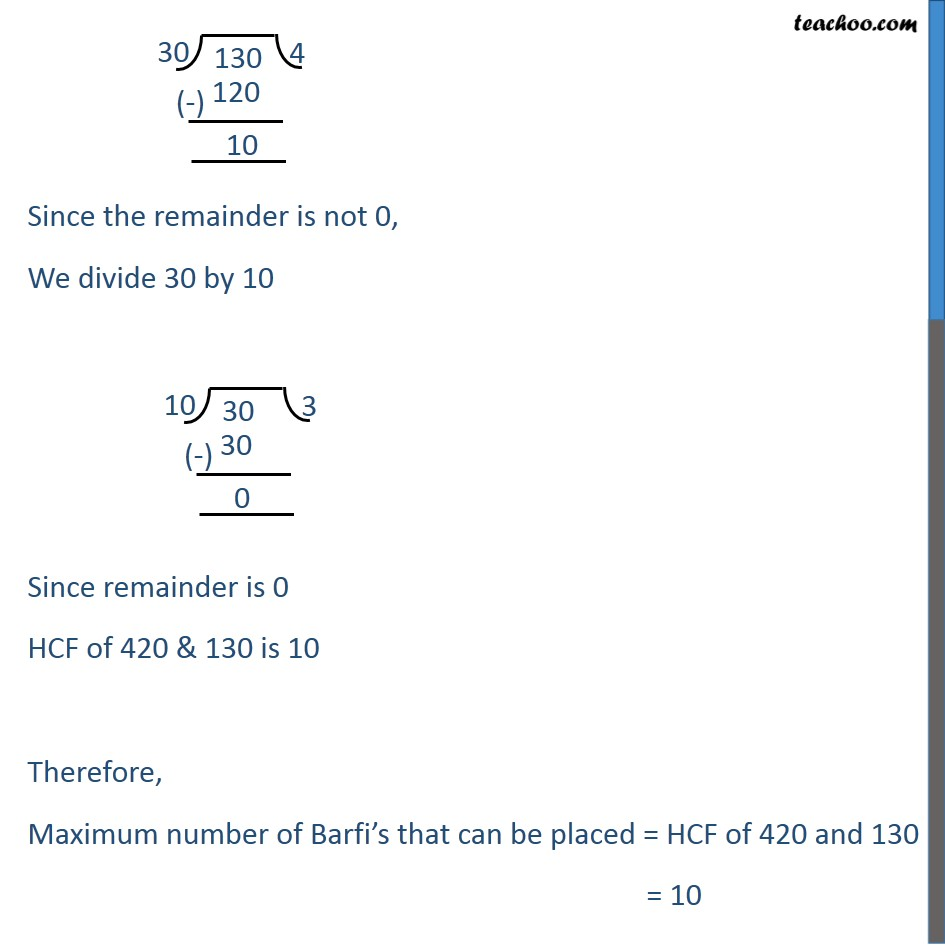Examples

Chapter 1 Class 10 Real Numbers
Serial order wiseLearn in your speed, with individual attention - Teachoo Maths 1-on-1 Class

### Transcript

Question 4 (Introduction) A sweet seller has 420 kaju barfis and 130 badam barfis. She wants to stack them in such a way that each stack has the same number, and they take up the least area of the tray. What is the maximum number of barfis that can be placed in each stack for this purpose? Suppose we have to stack 12 kaju barfis & 4 badam barfis Maximum number of barfis to stack = 4 = HCF of 12 & 4 Similarly , we will do in this question Question 4 A sweet seller has 420 kaju barfis and 130 badam barfis. She wants to stack them in such a way that each stack has the same number, and they take up the least area of the tray. What is the maximum number of barfis that can be placed in each stack for this purpose? Maximum number of Barfi’s that can be placed = HCF of 420 and 130 Using Euclid’s Division Algorithm Since 420 > 130 We divide 420 by 130 Since the remainder is not 0, We divide 130 by 30 Since the remainder is not 0, We divide 30 by 10 Since remainder is 0 HCF of 420 & 130 is 10 Therefore, Maximum number of Barfi’s that can be placed = HCF of 420 and 130 = 10